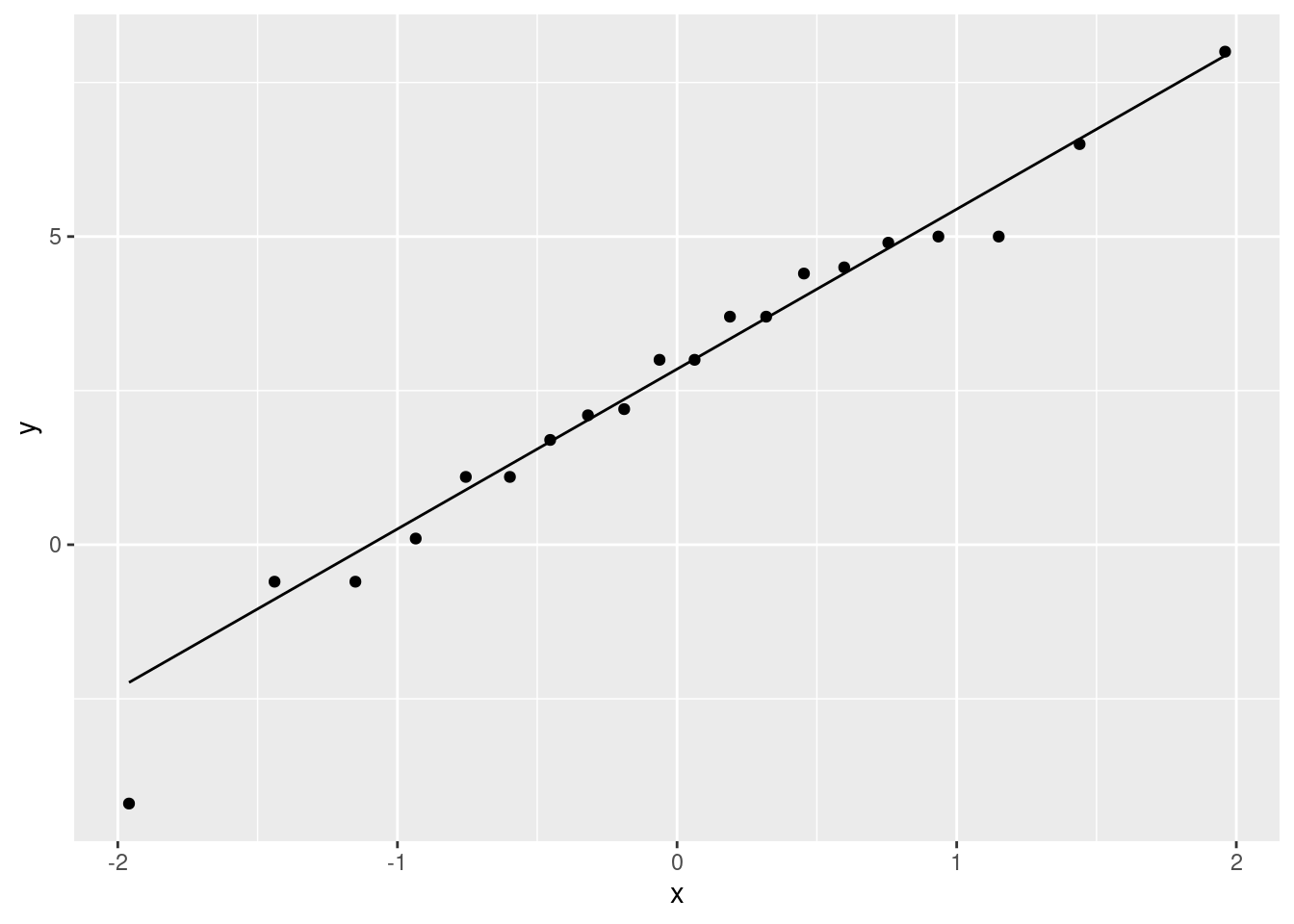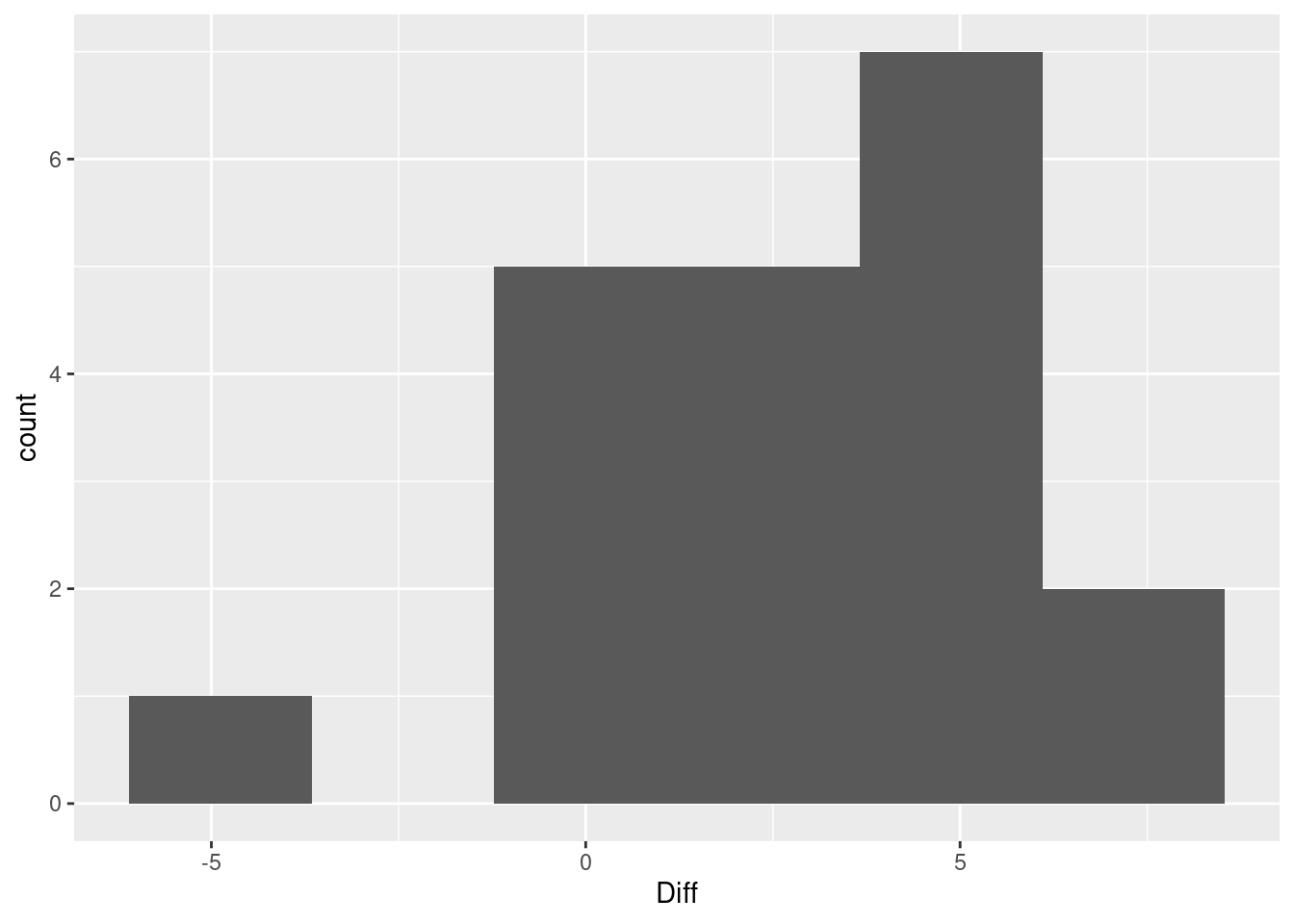# Worksheet 8

Published

October 17, 2023

Questions are below. My solutions are below all the question parts for a question; scroll down if you get stuck. There is extra discussion below that for some of the questions; you might find that interesting to read, maybe after tutorial.

For these worksheets, you will learn the most by spending a few minutes thinking about how you would answer each question before you look at my solution. There are no grades attached to these worksheets, so feel free to guess: it makes no difference at all how wrong your initial guess is!

# 1 Fuel efficiency comparison

Some cars have on-board computers that calculate quantities related to the car’s performance. One of the things measured is the fuel efficiency, that is, how much gasoline the car uses. On an American car, this is measured in miles per (US) gallon. On one type of vehicle equipped with such a computer, the fuel efficiency was measured each time the gas tank was filled up, and the computer was then reset. Twenty observations were made, and are in http://ritsokiguess.site/datafiles/mpgcomparison.txt. The computer’s values are in the column Computer. The driver also calculated the fuel efficiency by hand, by noting the number of miles driven between fill-ups, and the number of gallons of gas required to fill the tank each time. The driver’s values are in Driver. The final column Diff is the difference between the computer’s value and the driver’s value for each fill-up. The data values are separated by tabs.

1. Read in and display (some of) the data.

2. What is it that makes this paired data? Explain briefly.

3. Draw a suitable graph of these data, bearing in mind what you might want to learn from your graph.

4. Is there any difference between the average results of the driver and the computer? (Average could be mean or median, whichever you think is best). Do an appropriate test.

5. The people who designed the car’s computer are interested in whether the values calculated by the computer and by the driver on the same fill-up are usually close together. Explain briefly why it is that looking at the average (mean or median) difference is not enough. Describe what you would look at in addition, and how that would help.

My solutions

Some cars have on-board computers that calculate quantities related to the car’s performance. One of the things measured is the fuel efficiency, that is, how much gasoline the car uses. On an American car, this is measured in miles per (US) gallon. On one type of vehicle equipped with such a computer, the fuel efficiency was measured each time the gas tank was filled up, and the computer was then reset. Twenty observations were made, and are in http://ritsokiguess.site/datafiles/mpgcomparison.txt. The computer’s values are in the column Computer. The driver also calculated the fuel efficiency by hand, by noting the number of miles driven between fill-ups, and the number of gallons of gas required to fill the tank each time. The driver’s values are in Driver. The final column Diff is the difference between the computer’s value and the driver’s value for each fill-up. The data values are separated by tabs.

1. Read in and display (some of) the data.

Solution

library(tidyverse)

This is like the athletes data, so read_tsv:

my_url <- "http://ritsokiguess.site/datafiles/mpgcomparison.txt"
fuel <- read_tsv(my_url)
Rows: 20 Columns: 4
── Column specification ────────────────────────────────────────────────────────
Delimiter: "\t"
dbl (4): Fill-up, Computer, Driver, Diff

ℹ Use spec() to retrieve the full column specification for this data.
ℹ Specify the column types or set show_col_types = FALSE to quiet this message.
fuel

20 observations, with columns as promised. The first column Fill-up labels the fill-ups from 1 to 20, in case we needed to identify them. (This is an additional clue that we have paired data, or repeated measures in general.1)

See the Extra for another way to read in these data (and why it works).

$$\blacksquare$$

1. What is it that makes this paired data? Explain briefly.

Solution

There are two measurements for each fill-up: the computer’s calculation of gas mileage, and the driver’s. These go together because they are two calculations of what is supposed to be the same thing. (Another hint is that we have differences, with the veiled suggestion that they might be useful for something.)

If you want to have a general way of tackling this kind of problem, ask yourself “is there more than one observation of the same thing per individual?” Then go on and talk about what the individuals and observations are for the data you’re looking at. In this case, the individuals are fill-ups, and there are two observations per fill-up: one by the driver and one by the computer. Compare this with the children learning to read (from lecture): there were $$23+21 = 44$$ children altogether (individuals), and each child had one reading score, based on the reading method they happened to be assigned to. So those are 44 independent observations, and a two-sample test is the way to go for them.

$$\blacksquare$$

1. Draw a suitable graph of these data, bearing in mind what you might want to learn from your graph.

Solution

In a matched pairs situation, what matters is whether the differences have enough of a normal distribution. The separate distributions of the computer’s and driver’s results are of no importance. So make a graph of the differences. We are specifically interested in normality, so a normal quantile plot is best:

ggplot(fuel, aes(sample = Diff)) + stat_qq() + stat_qq_line()The next best plot is a histogram of the differences:

ggplot(fuel, aes(x = Diff)) + geom_histogram(bins = 6)This one actually looks left-skewed, or has an outlier at the bottom. The appearance may very well depend on the number of bins you choose.

$$\blacksquare$$

1. Is there any difference between the average results of the driver and the computer? (Average could be mean or median, whichever you think is best). Do an appropriate test.

Solution

The choices are a matched-pairs $$t$$-test, or a matched-pairs sign test (on the differences). To choose between those, look back at your graph. My take is that the only possible problem is the smallest (most negative) difference, but that is not very much smaller than expected. This is especially so given the sample size (20), which means that the Central Limit Theorem will help us enough to take care of the small outlier.

I think, therefore, that a paired $$t$$-test is the way to go, to test the null that the mean difference is zero (against the two-sided alternative that it is not zero, since we were looking for any difference). There are two ways you could do this: as literal matched pairs:

with(fuel, t.test(Computer, Driver, paired = TRUE))

Paired t-test

data:  Computer and Driver
t = 4.358, df = 19, p-value = 0.0003386
alternative hypothesis: true mean difference is not equal to 0
95 percent confidence interval:
1.418847 4.041153
sample estimates:
mean difference
2.73 

or, as a one-sample test on the differences:

with(fuel, t.test(Diff, mu = 0))

One Sample t-test

data:  Diff
t = 4.358, df = 19, p-value = 0.0003386
alternative hypothesis: true mean is not equal to 0
95 percent confidence interval:
1.418847 4.041153
sample estimates:
mean of x
2.73 

with the same result.

If you thought that low value was too much of an outlier, the right thing would have been a sign test that the median difference is zero (vs. not zero), thus (using the smmr package):

sign_test(fuel, Diff, 0)
$above_below below above 3 17$p_values
alternative     p_value
1       lower 0.999798775
2       upper 0.001288414
3   two-sided 0.002576828

In any of those cases, we conclude that the average difference is not zero, since the P-values are less than 0.05. (The right one for the sign test is 0.0026.)

I don’t mind which test you do, as long as it is one of the ways of doing matched pairs, and you justify your choice by going back to your graph. Doing one of the tests without saying why is being a STAB22-level statistician (in fact, it may not even be that), not a professional-level applied statistician.

$$\blacksquare$$

1. The people who designed the car’s computer are interested in whether the values calculated by the computer and by the driver on the same fill-up are usually close together. Explain briefly why it is that looking at the average (mean or median) difference is not enough. Describe what you would look at in addition, and how that would help.

Solution

The mean (or median) difference can be close to zero without the individual differences being close to zero. The driver could be sometimes way higher and sometimes way lower, and the average difference could come out close to zero, even though each individual pair of measurements is not that close together. Thus looking only at the average difference is not enough.

There are (at least) two (related) things you might choose from to look at, in addition to the mean or median:

• the spread of the differences (the SD or inter-quartile range). If the average difference and the spread of differences are both close to zero, that would mean that the driver’s and computer’s values are consistently similar.
• a suitable confidence interval here (for the mean or median difference, as appropriate for what you did) would also get at this point.

To think about the spread: use the SD if you did the $$t$$-test for the mean, and use the IQR if you did the sign test for the median, that is, one of these:

fuel %>% summarize(diff_sd = sd(Diff))

or

fuel %>% summarize(diff_iqr = IQR(Diff))

as appropriate. Make a call about whether you think your measure of spread is small or large. If you think it’s small, you can say that the differences are consistent with each other, and therefore that the computer’s and driver’s values are typically different by about the same amount (we concluded that they are different). If you think your measure of spread is large, then the driver’s and computer’s values are inconsistently different from each other (which would be bad news for the people who designed the car’s computer, as long as you think the driver was being reasonably careful in their record-keeping).

If you said that the confidence interval for the mean/median was the thing to look at, then pull the interval out of the $$t$$-test output or run ci_median, as appropriate. The computer’s miles per gallon is consistently between 1.4 and 4.0 miles per gallon higher (mean) or 1.1 and 4.5 miles per gallon higher (median).